# Estimation Techniques

#### Estimation is about finding an answer to a problem that is close enough to the exact answer.

In many real life situations calculating an approximate answer will be faster and easier and this may be more important than having a high degree of accuracy.

For example, if you are checking you have been charged the correct amount in a shop or working out your fair share of the bill in a restaurant, you may not be concerned about the pennies but you would want to pay the right amount in pounds.

We often rely on computers or calculators to make precise calculations for us but human error when using machines can lead to a precise but incorrect answer. In these situations, having a feel for the numbers, which comes from having good estimation skills, is important for checking that the answer is about the right size.

### Simplifying Calculations

When estimating, we replace numbers in a calculation with other numbers which are much easier to work with. We can do this by rounding each number either to the nearest whole number, 10, 100, 1000 etc or by rounding to a given number of significant figures. The more detail we keep in our numbers when we round, the more accurate our answer will be, but of course the more difficult the calculation will be.

A good approach is to round off all the numbers in a calculation to 1 significant figure. We can then work out our answer using our easy numbers.

We use the symbol ‘≈’ which means ‘approximately equal to’.

#### Example 1

4658 × 0.314

Solution

If we round off these values to 1 sig. fig.

4698 ≈ 5000 and 0.314 ≈ 0.3

So 4658 × 0.314 ≈ 5000 × 0.3 = 1500

#### Example 2

207.56 ÷ 397.63

Solution

If we round off these values to 1 sig. fig.

207.56 ≈ 200 and 397.63 ≈ 400

207.56 ÷ 397.63 ≈ 200 ÷ 400 = 0.5

#### Example 3

A kitchen is 8.9m wide and 11.4m long, and it will cost £7.85 per square metre for new flooring. Peter estimates that it will cost roughly £8000, and Paul estimates that it is nearer £800. Whose estimate is better?

Solution

If we round our measurement to the nearest whole metre,

8.9m ≈ 9m  and  11.4m ≈ 11m.

So the area of the room ≈ 9m × 11m = 99 square metres

Let’s say this is approximately 100 square metres

Rounding the cost of flooring to the nearest pound gives £8 per square metre.

The total cost will be in the region of £8 × 100 = £800.

Paul’s estimate is better. Peter’s estimate is ten times too big, we could say that it is the wrong order of magnitude.

#### Example 4

You have carried out a scientific experiment and the result is given by the formula below. The person you are working with has a calculator and uses it to get the answer 159.121, but you aren’t sure if they used their calculator correctly. How can you use estimation techniques to decide whether you accept their answer or not?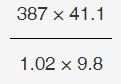Solution

Replacing each value with an approximate value gives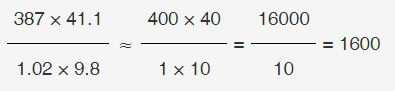Your estimate is about 10 times bigger than the value from the calculator. It would be a good idea to ask your friend to retry the calculation or ask to use the calculator yourself.

### Dealing with Very Small Numbers

When adding or subtracting, very small numbers can be approximated by zero.

#### Example 5

1999 + 0.0001

Solution

1999 + 0.0001 ≈ 2000 + 0 = 2000

#### Example 6

389 – 0.034

Solution

389 – 0.034 ≈ 400 – 0 = 400

When multiplying or dividing, you must never approximate a small number with zero. Round the number to 1 significant figure or replace with 0.1, 0.01, 0.001 etc.

#### Example 7

0.0099 × 813

Solution

If we round these values to 1 sig. fig.

0.0099 × 813 ≈ 0.01 × 800 = 8

If we had approximated with zero here, we would have got the answer zero.

#### Example 8

5 ÷ 0.00879

Solution

If we round 0.00879 to 0.01 then

5 ÷ 0.00879 ≈ 5 ÷ 0.01 = 500

If we had approximated with zero here, we would have got 5 ÷ 0, which can’t be calculated. If you try this on your calculator, you will find you get an error message.

### Exercise 1

In a café you order 3 Lattes at £2.15 a Cappuccino at £2.05 and 2 teas at £1.85. You pay with a £20 note and get £5.75 in change. Have you been given the correct amount?

#### Solution

All the drinks cost about £2 and you have ordered 6 drinks. The total cost should be around £12 and you would expect about £8 in change. You ask the barista to check the order and it turns out you have been charged for 2 Cappuccinos instead of 1. You are given the correct amount of change which is £7.80.

### Exercise 2

You are calculating 245 × 48 on your calculator and you get the answer 1200. Is this the right answer?

#### Solution

245 × 48 is a bit less than 250 × 50 which is 12 500.

You must have typed something wrong on your calculator. In fact, you probably typed 25 × 48 which is 1200. Missing one digit on the calculator could have led to an incorrect answer.

### Exercise 3

A baguette costs £1.95 at the café and there are 9 of us in the office who would like a baguette. How much money will I need to take to the shop?

#### Solution

Round up the price of a baguette to £2, and the number of people to 10.

The estimated cost will be: £2 × 10 = £20.

Since we overestimated the cost and the number of people, the cost will be less than £20.

Taking a £20 note to the shop will be enough.

### Exercise 4

Homer brings home a bonus of \$102.50. He wants to divide it equally between himself, Marge, Bart, Lisa, and Maggie. He calculates that he can give each family member \$15.20. Lisa protests that he is short-changing them. Roughly how much should each person expect to receive?

#### Solution

Lisa is right of course!

Homer’s bonus ≈ \$100.

Divided by 5 people this gives \$100 ÷ 5 = \$20.

Since we slightly underestimated Homer’s bonus, each family member should get just over \$20.

### Exercise 5

100 + 0.00031

#### Solution

100 + 0.00031 ≈ 100 + 0 = 100

### Exercise 6#### Solution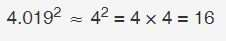### Exercise 7

987.9 – 0.03

#### Solution

987.9 – 0.03 ≈ 988 – 0 = 988

### Exercise 8

0.098 × 24

#### Solution

0.098 × 24 ≈ 0.1 × 24 = 2.4

### Exercise 9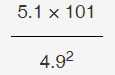#### Solution### Exercise 10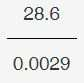#### Solution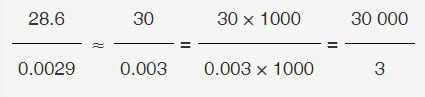= 10 000

### Exercise 11

251 ÷ 0.0095

#### Solution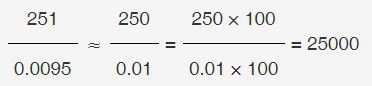### Exercise 12

48965 × 0.00638

#### Solution

48965 × 0.00638 ≈ 50000 × 0.006

= 50 × 6 = 300